# Expression of a variable from the formula + triangle - math problems

#### Number of problems found: 388

• An equilateral triangle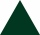The perimeter of an equilateral triangle is 33cm. How long is each side?
• Chord of triangle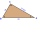If the whole chord of the triangle is 14.4 cm long, how do you calculate the shorter and longer part?
• Isosceles triangleThe circumference of the isosceles triangle is 32.5 dm. Base length is 153 cm. How long is the leg of this triangle?
• Equilateral triangleCalculate the area of an equilateral triangle with circumference 72cm.
• Isosceles right triangleArea of an isosceles right triangle is 18 dm2. Calculate the length of its base.
• Three altitudes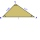A triangle with altitudes 4; 5, and 6 cm is given. Calculate the lengths of all medians and all sides in a triangle.
• Right triangleA circle with a radius of 5 cm is described in a right triangle with a 6 cm leg. What is the height at the hypotenuse of this triangle?
• CalculateCalculate the length of a side of the equilateral triangle with an area of 50cm2.
• In aIn a triangle, the aspect ratio a: c is 3: 2 and a: b is 5: 4. The perimeter of the triangle is 74cm. Calculate the lengths of the individual sides.
• Largest angle of the triangleCalculate the largest angle of the triangle whose sides have the sizes: 2a, 3/2a, 3a
• Isosceles triangle 10In an isosceles triangle, the equal sides are 2/3 of the length of the base. Determine the measure of the base angles.
• Medians in RT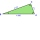The rectangular triangle ABC has a length of 10 cm and 24 cm. Points P, Q, R are the centers of the sides of this triangle. The perimeter of the PQR triangle is:
• Right-angled triangle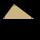Determine the content of a right triangle whose side lengths form successive members of an arithmetic progression and the radius of the circle described by the triangle is 5 cm.
• Triangle KLMIn the rectangular triangle KLM, where is hypotenuse m (sketch it!) find the length of the leg k and the height of triangle h if hypotenuse's segments are known mk = 5cm and ml = 15cm
• Equilateral triangleThe equilateral triangle has a 23 cm long side. Calculate its content area.
• Triangle perimeterCalculate the triangle perimeter whose sides are in ratio 3: 5: 7 and the longest side is 17.5 cm long.
• Inner anglesThe magnitude of the internal angle at the main vertex C of the isosceles triangle ABC is 72°. The line p, parallel to the base of this triangle, divides the triangle into a trapezoid and a smaller triangle. How big are the inner angles of the trapezoid?
• Rectangular triangleThe lengths of the rectangular triangle sides with a longer leg of 12 cm form an arithmetic sequence. What is the area of the triangle?
• Right triangleLegs of the right triangle are in the ratio a:b = 2:8. The hypotenuse has a length of 87 cm. Calculate the perimeter and area of the triangle.
• The angles ratioThe angles in the ABC triangle are in the ratio 1: 2: 3. find the sizes of the angles and determine what kind of a triangle it is.

Do you have an interesting mathematical word problem that you can't solve it? Submit a math problem, and we can try to solve it.

We will send a solution to your e-mail address. Solved examples are also published here. Please enter the e-mail correctly and check whether you don't have a full mailbox.

Please do not submit problems from current active competitions such as Mathematical Olympiad, correspondence seminars etc...